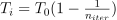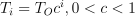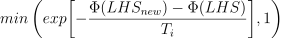# Optimize an LHS design of experiments¶

This examples show how to generate optimized LHS experiments according to the different criteria.

import openturns as ot
import openturns.viewer as viewer
from matplotlib import pylab as plt

ot.Log.Show(ot.Log.NONE)


LHS and space filling

N = 100
# Considering independent Uniform distributions of dimension 3
# Bounds are (-1,1), (0,2) and (0, 0.5)
distribution = ot.ComposedDistribution(
[ot.Uniform(-1.0, 1.0), ot.Uniform(0.0, 2.0), ot.Uniform(0.0, 0.5)]
)
# Random LHS
lhs = ot.LHSExperiment(distribution, N)
lhs.setAlwaysShuffle(True)  # randomized
design = lhs.generate()
# C2
c2 = ot.SpaceFillingC2().evaluate(design)
# PhiP with default p
phip = ot.SpaceFillingPhiP().evaluate(design)
# mindist
mindist = ot.SpaceFillingMinDist().evaluate(design)
# For p->infinity
phip_inf = ot.SpaceFillingPhiP(100).evaluate(design)
print(phip, mindist, phip_inf)

17.054824704132752 0.0469316649638053 17.054817010338425


Optimized LHS using Monte Carlo

As with Monte Carlo, user decides of a fixed number of iterations, but this time this number is part of the temperature profile.

Two profiles are currently provided: - Linear profile:- Geometric profile:Starting from an LHS design, a new design is built by permuting a random coordinate of two randomly chosen sample points; this new design is also an LHS. but not necessary a more efficient design.

A comparison of criteria of the two designs is done, and the new LHS is accepted with probabilityConsidering independent Uniform(0,1) distributions of dimension 3

distribution = ot.ComposedDistribution([ot.Uniform(0.0, 1.0)] * 3)
# Random LHS
lhs = ot.LHSExperiment(distribution, N)
lhs.setAlwaysShuffle(True)  # randomized
algo = ot.SimulatedAnnealingLHS(lhs)
design = algo.generate()


One could also fix the criterion, the temperature profile and get more results.

# Considering independent Uniform distributions of dimension 3
# Bounds are (-1,1), (0,2) and (0, 0.5)
distribution = ot.ComposedDistribution(
[ot.Uniform(-1.0, 1.0), ot.Uniform(0.0, 2.0), ot.Uniform(0.0, 0.5)]
)
# Random LHS
lhs = ot.LHSExperiment(distribution, N)
lhs.setAlwaysShuffle(True)  # randomized
# Fixing C2 crit
space_filling = ot.SpaceFillingC2()
# Defining a temperature profile
# A geometric profile seems accurate with default parameters
# e.g. T0=10, c=0.95, iMax=2000
temperatureProfile = ot.GeometricProfile()
algo = ot.SimulatedAnnealingLHS(lhs, space_filling, temperatureProfile)
# optimal design
design = algo.generate()
result = algo.getResult()
# Criteria for the optimal design
crit_c2 = result.getC2()
crit_phip = result.getPhiP()
crit_mindist = result.getMinDist()
# History of the criterion used for optimization
history = result.getAlgoHistory()
criterion_hist = history[:, 0]
temperature_hist = history[:, 1]
probability_hist = history[:, 2]


It is also possible to chain several iterations of the whole process with different starting points.

N = 10

# Considering independent Uniform distributions of dimension 3
# Bounds are (-1,1), (0,2) and (0, 0.5)
distribution = ot.ComposedDistribution(
[ot.Uniform(-1.0, 1.0), ot.Uniform(0.0, 2.0), ot.Uniform(0.0, 0.5)]
)
# Random LHS
lhs = ot.LHSExperiment(distribution, N)
lhs.setAlwaysShuffle(True)  # randomized
# Fixing PhiP crit
space_filling = ot.SpaceFillingPhiP()
# Defining a temperature profile
# T0=10, iMax=3000
temperatureProfile = ot.LinearProfile(10.0, 3000)
algo = ot.SimulatedAnnealingLHS(lhs, space_filling, temperatureProfile)
restart = 50
design = algo.generateWithRestart(restart)
# Retrieve all optimal designs
result = algo.getResult()
designs = [result.getOptimalDesign(i) for i in range(restart)]


Finally, we could start the optimization process of LHS using a precomputed LHS design.

# Considering independent Uniform distributions of dimension 3
# Bounds are (0,1)^3
distribution = ot.ComposedDistribution([ot.Uniform(0.0, 1.0)] * 3)
# Random LHS
lhs = ot.LHSExperiment(distribution, N)
lhs.setAlwaysShuffle(True)  # randomized
# Fixing C2 crit for example
space_filling = ot.SpaceFillingC2()
# Defining a temperature profile
# T0=10, iMax=3000
temperatureProfile = ot.LinearProfile(10.0, 3000)
algo = ot.SimulatedAnnealingLHS(lhs, space_filling, temperatureProfile)
design = algo.generate()
result = algo.getResult()
# check history ==> draw criterion
graph = result.drawHistoryCriterion()
view = viewer.View(graph)Convergence needs to be performed New algo starting from this design

algo = ot.SimulatedAnnealingLHS(design, distribution, space_filling, temperatureProfile)
design = algo.generate()
plt.show()